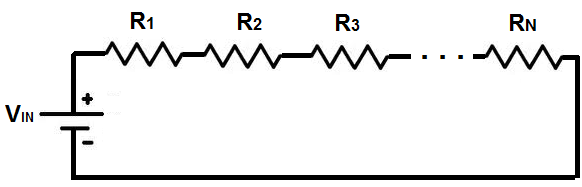﻿ Voltage Divider Calculator ﻿# Voltage Divider Calculator

### Voltage Divider Between 2 ResistorsYou must enter values into all 3 form fields to compute result.

 VIN V R1 Ω (ohms) KΩ (Kilohms) MΩ (Megohms) R2 Ω (ohms) KΩ (Kilohms) MΩ (Megohms)

VOUT=

The voltage divider calculator calculates the output voltage of the voltage divider network based on the value of resistor, R1, resistor, R2, and the input voltage, VIN. This output voltage, which is the voltage that is dropped across resistor, R2, is calculated by the formula, VOUT= VIN (R2/(R1 + R1)).

According to this formula, the resistor with the greater resistance value will drop more voltage across it; and, conversely, the resistor with the smaller resistance value will drop less voltage across it.

To use this voltage divider calculator, a user must enter the value of the input voltage, VIN, the value of resistor R1, and the value of resistor, R2, and click the 'Calculate' button. The voltage output result will then be calculated and automatically displayed. The result of this output voltage is calculated in unit volts (V).

Voltage dividers are very common and used very often in circuits. Many times in circuits, different levels of voltage must be allocated to different parts of a circuit. To do this, a voltage divider circuit, such as that shown above, can be used. This can divide the input voltage that a circuit receives and allocate it accordingly and as needed to different parts of the circuit. For example, a circuit may receive 10V as input. However, one chip in the circuit may need 7 volts, while another chip in the circuit only needs 3 volts. We can allocate these voltages to the different chips by a voltage divider. If we make one resistor have a value of 3KΩ and the other resistor have a value of 7KΩ, this will allocate the 10 volts into 3V and 7V.

Example Calculation

If resistor R1 is 1KΩ and resistor R2 is 2KΩ and the input voltage, VIN is 12V, what is the output voltage, VOUT?

VOUT= VIN(R2/(R1 + R2)= (12V)(2KΩ)/(1KΩ + 2KΩ)= 8V.

So VOUT= 8 volts.

### Voltage Divider With Multiple Resistors

Up to 10 ResistorsVIN: V R1: Ω (ohms) KΩ (Kilohms) MΩ (Megohms) R2 Ω (ohms) KΩ (Kilohms) MΩ (Megohms) R3 Ω (ohms) KΩ (Kilohms) MΩ (Megohms) R4 Ω (ohms) KΩ (Kilohms) MΩ (Megohms) R5 Ω (ohms) KΩ (Kilohms) MΩ (Megohms) R6 Ω (ohms) KΩ (Kilohms) MΩ (Megohms) R7 Ω (ohms) KΩ (Kilohms) MΩ (Megohms) R8 Ω (ohms) KΩ (Kilohms) MΩ (Megohms) R9 Ω (ohms) KΩ (Kilohms) MΩ (Megohms) R10 Ω (ohms) KΩ (Kilohms) MΩ (Megohms)

This voltage divider calculator allows a user to calculate the voltage across several resistors in series with a DC voltage source (up to 10 resistors).

This voltage divider works by calculating up the total resistance in series of the entire circuit. It then uses the voltage divider formula of, Voltage Drop Across Resistor 1= (Voltage Input)(Resistance Value of Resistor 1)/(Total Resistance in Series), where Resistor 1 is the resistance of the individual resistor for which we want to find the voltage drop across. This formula is repeated for all resistors in the voltage divider circuit.

As ohm's law follows, more voltage will drop across the elements with the higher resistance values. And conversely, less voltage falls across resistors with lower resistance values.

To use this voltage divider calculator (for multiple resistors in series), a user must enter the value of the input voltage, VIN, and can enter in the resistor values of anywhere from 1 to 10 resistors and click the 'Calculate' button. The voltage drop across each of the resistors entered in will be computed and automatically displayed. The result of this voltage is calculated in unit volts (V).

The user can enter anywhere from 1 to 10 resistors. For example, if you would just like to measure the voltage drop across 3 resistors, then you would just enter in the value of the DC voltage source and the 3 resistor values and leave the rest of the fields blank. The calculator will compute the value of the voltage drops across these 3 resistors. If you want to calculate the voltage drop across 8 resistors, then you would just add the resistor values in those 8 fields and leave the remaining 2 fields blank. And it can go all the way up to all 10 resistors. This calculator can calculate down to 1 resistor. If you just add in 1 resistor, the voltage drop across that resistor will be equal to the DC voltage source (since the voltage isn't divided up between multiple resistors). Technically, this is not a voltage divider circuit, but the calculator will still compute it.

This second voltage divider calculator is superior to the first one. This is because it can calculate any voltage divider circuit from 2 to 10 resistors. Thus, the first calculator, technically, isn't needed. However, I have included it because a voltage divider between 2 resistors like that is a very commonly seen circuit and most people are familiar with the formula shown. Nonetheless, this second calculator can make the same computation as the first and more.

Example Calculation

If resistor R1 is 1KΩ and resistor R2 is 1KΩ and R3 is 1KΩ and the input voltage, VIN is 15V, what are the voltage drops across these 3 resistors.

VOUT across R1= VIN(R1/(R1 + R2 + R3)= (15V)(1KΩ)/(1KΩ + 1KΩ + 1KΩ )= 5V

VOUT across R2= VIN(R2/(R1 + R2 + R3)= (15V)(1KΩ)/(1KΩ + 1KΩ + 1KΩ )= 5V

VOUT across R3= VIN(R3/(R1 + R2 + R3)= (15V)(1KΩ)/(1KΩ + 1KΩ + 1KΩ )= 5V

Since all resistors are the same value, the voltage drops across each of them are the same.

Related Resources

﻿